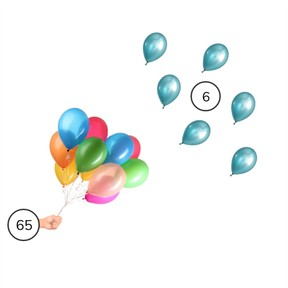Subtraction to 100 regrouping with subtrahends <10

# Subtraction to 100 regrouping with subtrahends <108,000 schools use Gynzy92,000 teachers use Gynzy1,600,000 students use Gynzy

## General

Students learn to subtract numbers to 100 regrouping with subtrahends less than 10.

2.NBT.B.5
2.OA.A.1

## Relevance

It is important to be able to subtract numbers to 100, so you can determine how much you have left.

## Introduction

Ask students to determine how many leaves have fallen from the tree. Example: There are 44 leaves on the tree and 7 fall off. How many leaves are left on the tree?

## Development

Discuss the importance of subtracting numbers to 100. The learning goal will be presented in three ways, as visual, abstract and story problems. You can use the blue menu in the bottom right to select between these ways to choose the one that best suits your classroom needs.
For the visual method, start by counting all of the objects. Next explain to students how to take the second number away from the first. Show that you first count back from 64 to the previous tens number. So you take 4 away to reach 60. Then you take away the rest, so take 3 away. The difference is 57. Solve the next problem together with the students. Check that students are able to subtract using visual support by using the next subtraction problems. Next students are shown how to solve in the abstract, using a number line. Explain to students how to show subtraction as jumps on the number line. Tell students that they also jump to the previous tens number first on the number line. The next problem is abstract without a number line. Check that students are able to subtract numbers without using the number line by asking them to solve the given subtraction problems. Ask students to explain their strategy. Emphasize that there are different ways to subtract and that students should use the one that they find easiest to use. Finally in story form, explain to students what steps they should take to solve a story problem- determine what kind of math problem it is, determine which numbers are required for the math problem, to say or write the problem and then to solve the problem. Practice a story problem as a class and then have students practice individually or in pairs.

Check that students are able to solve subtraction problems to 100 regrouping with subtrahends less than ten by asking the following questions:
- Why is it useful to be able to subtract numbers to 100?
- How do you calculate a subtraction problem with numbers to 100?

## Guided practice

Students are given subtraction problems with visual support, as abstract problems, and in story form.

## Closing

Check that students have understood the learning goal by asking them how they can solve a subtract problem with numbers to 100. Emphasize that each student might have a different way to solve their subtraction problem. To close the lesson, have students determine if the subtraction problems shown have been solved correctly or incorrectly. Erase the grey square at the end to check if it is correct or incorrect. Then ask students to drag the differences so that each subtraction problem is correct.

## Teaching tips

Students who have difficulty subtracting with numbers to 100 can start by practicing subtraction problems to 50. They can use MAB-blocks or other manipulatives to help visualize the numbers. Help students regroup by having them split the subtrahend so they can step back to the previous tens number and then continue their subtraction. They can also be supported by making use of the number line.

## Instruction materials

Optional: MAB blocks or other manipulatives.

### The online teaching platform for interactive whiteboards and displays in schools

• Save time building lessons

• Manage the classroom more efficiently

• Increase student engagement